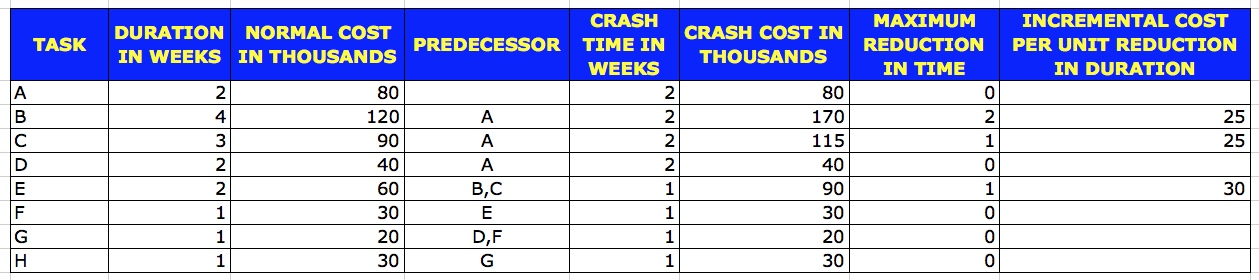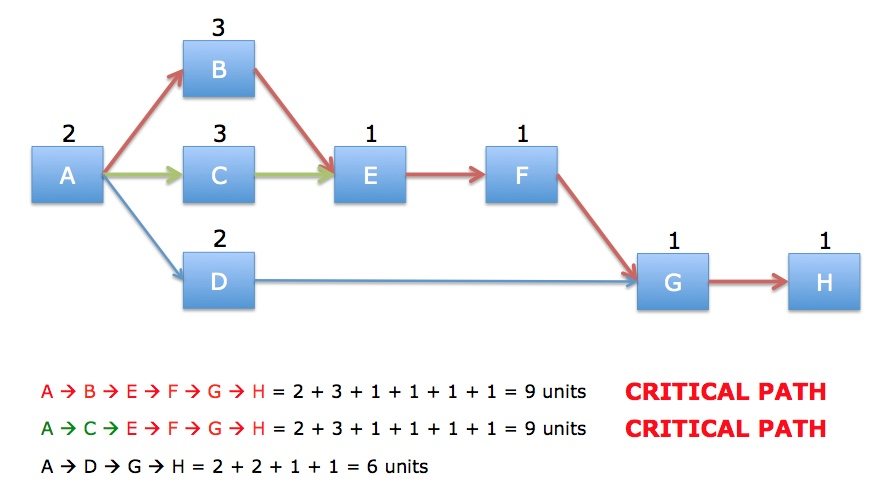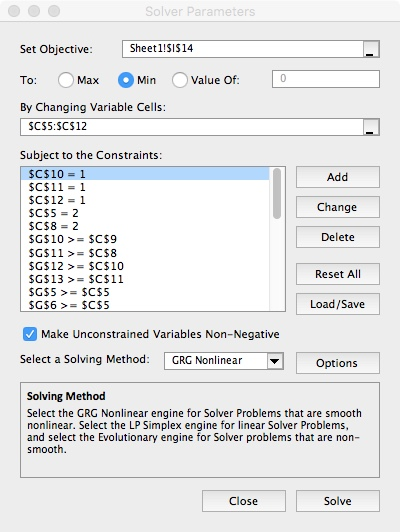# Applying Linear Programming to Project Management

Linear Programming has many applications in Project Management. I present you one such application. Once you get the idea, you can apply it in so many different scenarios.

One of the major requirement of any Project Manager is to be able to complete the project early. There can be many reason why one may need to complete the Project early. Some of the reasons could be

• Early realisation of revenues
• Reduction in Direct and Indirect Costs
• It may be due to Customer Requirement
• It may be due to Contract Commitments (There are usually bonus associated with early completion of projects).
• Time to Market Pressures
• Pressure to move the resources to other Projects
• etc.

However, trying to complete the Project Early comes with a cost. Typical examples of cost associated with trying to complete the Project early are

• Cost due to added resources to the Project
• Cost due to Overtime spent on the Project
• etc.
So one has to balance between the benefits of completing the project early with the increased direct cost of completing the activities.
There are several possible alternatives for reducing the completion time of the project. These include
• scheduling overtime
• outsourcing some project work
• forming a competent, dedicated, and focused core project team
• adopting a Critical-Chain approach to project management
• etc.

Consider the following Project Schedule.The network diagram for this Project Schedule is as shown below.With normal duration for each task, the project completion time is 11 weeks. The critical part is shown in red in the diagram.

Now we’ll consider the problem of reducing some of the task durations, in order to complete the project early. In order to reduce the duration of a task from its normal time, we have to incur incremental direct cost, consisting of perhaps overtime payment and our cost of additional resources. The relationship between the incremental data cost and the reduction in task time is assumed to be linear. For each task whose duration can be reduced, we need to estimate the incremental data cost for each unit reduction and duration.

For each task, there would be a minimum required time beyond which the task time cannot be reduced. The minimum time and the corresponding data cost are often referred to as crash time and crash cost. You can, if necessary, consider certain type of non-linear relationships between the reduction in the activity duration and the increase in direct cost but we will not do so in this example.
For our example, consider the following data. I have planted this data in a Excel Workbook. For Linear Programming, any tool available could be used. However, I have used Excel as I expect most reader to have Excel available with them.The table shows the normal time and the associated normal direct cost, as well as the crash time and the associated crash cost. From this, we can calculate, as shown in the table, for each task, the maximum possible reduction from the normal duration and the slope of the assumed linear relationship.

Now suppose there’s a bonus of 40,000 for each duration reduction in project completion time by one week.

## First, let us try to solve this problem manually.

### We want to determine the least incremental cost for reducing the project completion time by one week at a time.

The project completion time can be reduced only if you reduce the duration of the tasks on the critical path, which are tasks A –> B –> E –> F –> G –> H.
In this critical path, the duration of only tasks B and D can be reduced. For task B, the incremental cost of reducing the duration by one unit is 25,000 while the incremental cost of reducing the duration of task E is 30,000. Hence, it is better to reduce the duration of task B.
Since the bonus is 40,000 for one week reduction in project completion time, we reduce the duration of the task by one unit. That is the duration of the task be reduced from 4 weeks to 3 weeks incurring an incremental cost of 25,000 while getting a bonus of 40,000. Thereby, having an net gain of 40000 – 25000 = 15000.
Now the project completion time is 10 weeks. We now have two critical paths as shown in the diagram below.In order to reduce a project completion time further, we need to reduce the length of each of the paths A –> B –> E –> F –> G –> H and A –> C –> E –> F –> G –> H. Now we have to consider two possibilities, one in which the duration of the task E that is common to both of the paths, is reduced by 1 unit. And the other in which we reduce the duration of two distinct tasks – B and C, one in each path where each task is in one and only one of the two critical paths.
An incremental cost of reducing of task E by one week is 30,000. The other possibility is reduce the duration of both tasks B and C by 1 week each, increment of direct cost of 25,000 plus 25,000, which is 50,000.
Since 30,000 is less than 50,000, and 30,000 is less than the bonus of 40,000, we reduce the duration of E by 1 week (i.e. from 2 weeks to 1 week), thereby having a net gain of 40000 – 30000 = 10000. Now the project completion time is 9 weeks.We still have the same two critical paths.

Now, since all tasks except B and C are at their minimum crash duration, the only possibility is to reduce the duration of both tasks B and C by 1 week.
This, however, implies that the increase in direct cost would be 50,000 for reducing project duration by 1 week. Since the bonus is only 40,000 per week reduction, we will not reduce the duration of tasks B and C.
If the bonus was at least 50,000 per week, we would reduce the duration of tasks B and C by 1 week and then the project completion time would be 8 weeks.
No further reduction and project completion time is possible since all the tasks in the critical path are at a their minimum duration.
It should be noted as you keep reducing the task durations, more paths become critical, and more tasks are on one or more critical paths. The critical tasks must be managed well so that none of them can take a longer time than the stipulated duration if the project completion time is not to be delayed. So, reducing the project completion time typically implies that the flexibility is lost and project management becomes more difficult.

## The above approach to reduce the product duration is conceptually sound. But for large problems, it becomes very cumbersome and difficult to implement. A Linear Programming approach to solving this problem would be most appropriate.

Using Excel, we can use the feature of the Add-In Solver to solve Liner Programming Problems.
To configure the Solver, we need 3 pieces – Objective Function which has to be maximised or minimised, Variables to be altered to meet the requirement of the Objective Function and Constraints which have to be considered for optimisation.
The Objective Function in this case is to minimise the total duration of the Project. So, in the Solver, point to the Cell where the calculation for the total duration of the project is stored.
The variables for this problem are the durations which have to be altered. In the Solver, configure the Cells where the Duration of the tasks are stored.
The Constraints are as follows:
1. We need the durations to remain non-negative as a solution. So, we add one constraint for each duration referring to the Cell Address > 0. For example, if we have store the duration for Task A in Cell C4, then one constraint becomes C4 > 0.
2. We need the durations to remain an Integer. So, we add one constraint for each duration variable. For example, if we have stored the duration for Task A in Cell C4, then one constraint becomes C4 is Integer.
3. We need the Precedences to remain honoured in the solution. If the start date of Task A is in Cell D4 and the start date of Task B is in Cell D5, then one constraint would be D5 – D4 >= 2. However, we cannot enter a formula in the Constraints area of Solver. So, we need to add a new column where we can store the differences like D5 – D4. Say this Cell is E5. Then we can add the constraint E5 >= 2.
4. We also need to add constraints to indicate that certain task durations cannot be altered. This can be done by fixing the duration of these tasks as per the current duration. For example, the duration of Task A cannot be crashed. So, we add a constraint as C4 = 2, where we assume that Cell C4 stores the duration for Task A and the duration for Task A is 2.Click on Solve to get the optimised result.

Categories: Technical

This site uses Akismet to reduce spam. Learn how your comment data is processed.